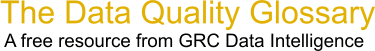Choose Index below for a list of all words and phrases defined in this glossary.

# Factoring

index | Index

## Factoring - definition(s)

factoring, prime numbers - Factoring and prime numbers are used in one of the most commonly used public key systems, the RSA system, to stifle attacks.

In asymmetric cryptography it's essential that an attacker cannot use one key, for example the public key, to find the value of the private key. In order to do this, a one-way, or trapdoor mathematical function is required. This is a mathematical function that's easy to do in one direction but is very difficult, or impossible to reverse. Factoring prime numbers is like this. While it is easy to find the product of two large prime numbers, finding the unique value of those two prime numbers, called factoring, is very difficult if all that you know is the product.

Very large prime numbers are used, because the larger the prime number, the more difficult factoring becomes.

[Category=Geospatial ]

Source: RSA, 15 August 2011 09:35:46, http://www.rsa.com/glossary/Data Quality Glossary.  A free resource from GRC Data Intelligence. For comments, questions or feedback: dqglossary@grcdi.nl